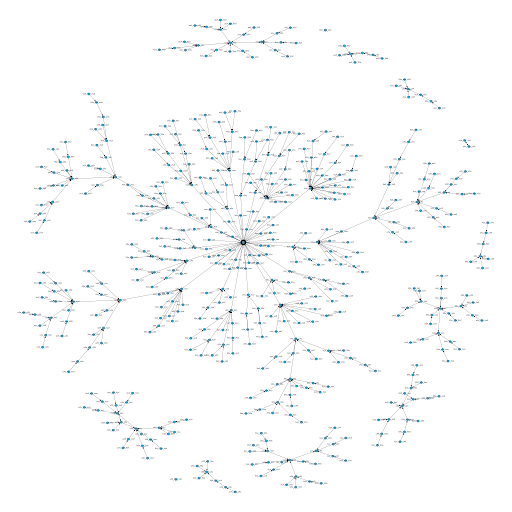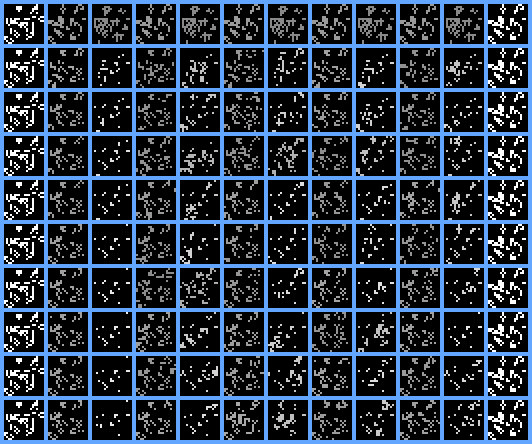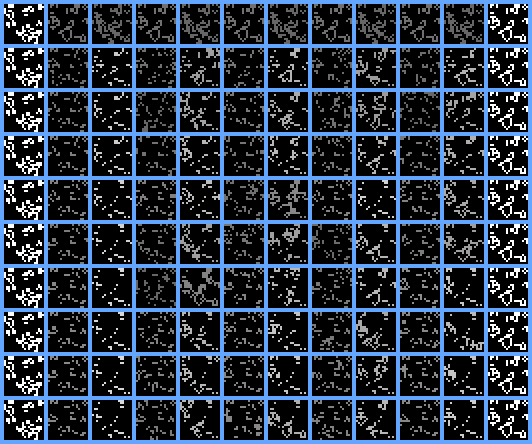# Diving into GoLang

5 June 2014

## Introduction

Who I am, what I'm doing this year

## Overview

• Introduction
• What is Kaggle?
• Kaggle Reverse-GoL Competition
• Benchmarking Stuff
• Revese-GoL Strategy: Genetic Algorithms
• Go : The Good Bits
• Go : The Bad Bits
• The Kaggle Thing
• End Result

## Kaggle Competitions## Standard Game-of-Life## Kaggle Reverse-GoL

• Standard Game-of-Life iterates board FORWARDS
• Kaggle competition is to do the REVERSE ::
• Given an ending board, what was the starting board {1, 2, 3, 4 or 5} steps ago?
• Each board is 20x20
• 50,000 board test-set

## Board Densities

Simple visualization of nature of problem## Training Examples

(also checking that board code works)Single 'delta' = 5

## Reverse GoL Issues• Information loss
• Scoring system
• Speed-of-light : delta=n -> 2^(nxn)

## Benchmarking

Speed of Board iteration is VERY IMPORTANTImage source: Weisstein, Eric W. "Game of Life."
From MathWorld -- A Wolfram Web Resource.

## Python : NumPy-style

"NumPy is C-Speed"

``````
import numpy

def iterate(Z):
# find number of neighbours that each square has
N = numpy.zeros(Z.shape)
N[1:, 1:] += Z[:-1, :-1]
N[1:, :-1] += Z[:-1, 1:]
N[:-1, 1:] += Z[1:, :-1]
N[:-1, :-1] += Z[1:, 1:]
N[:-1, :] += Z[1:, :]
N[1:, :] += Z[:-1, :]
N[:, :-1] += Z[:, 1:]
N[:, 1:] += Z[:, :-1]
# a live cell is killed if it has fewer
# than 2 or more than 3 neighbours.
part1 = ((Z == 1) & (N < 4) & (N > 1))
# a new cell forms if a square has exactly three members
part2 = ((Z == 0) & (N == 3))
return (part1 | part2).astype(int)

glider = numpy.array([[0,0,1],
[1,0,1],
[0,1,1]])

Z = numpy.zeros((22,22), dtype=numpy.int)
Z[1:1+glider.shape, 1:1+glider.shape] = glider
``````

Time for 65k timesteps : 8.7s

## Python : Bit-Twiddle-style

"Tell Python about C Kung-Fu"

``````

class BitField:  # Always surrounded by zeroes
bitarr = []

def __init_COUNT_BITS():
#print "__init_COUNT_BITS()"
arr=[]
for x in range(0, 1<<9):
cnt = bin(x).count('1')
arr.append(cnt)
return arr
COUNT_BITS = __init_COUNT_BITS()
del __init_COUNT_BITS

def __init__(self, n_rows=22, n_cols=22, numeric_array=None):
self.n_rows=n_rows # Includes the padded region
self.n_cols=n_cols # Includes the padded region
self.bitarr = [ 0 for i in range(0, self.n_rows) ]

def iterate(self):
## These are constants - the game bits pass over them
tb_filter  = 7
mid_filter = 5
current_filter = 2

arr_new=
for r in range(1, self.n_rows-1):
r_top = self.bitarr[r-1]
r_mid = self.bitarr[r]
r_bot = self.bitarr[r+1]

acc = 0
p = 2 # Start in the middle row, one column in

for c in range(1, self.n_cols-1):
cnt = self.COUNT_BITS[
((r_top & tb_filter) << 6 )|
((r_mid & mid_filter) << 3 )|
(r_bot & tb_filter)
]

#if True: acc |= p  # Check bit-twiddling bounds

# Return next state according to the game rules:
#  exactly 3 neighbors: on,
#  exactly 2 neighbors: maintain current state,
#  otherwise: off.
#  return alive == 3 || alive == 2 && f.Alive(x, y)
if (cnt==3) or (cnt==2 and (r_mid & current_filter)) :
acc |= p

# Move the 'setting-bit' over
p <<= 1

# Shift the arrays over into base filterable position
r_top >>= 1
r_mid >>= 1
r_bot >>= 1

arr_new.append(acc)
arr_new.append(0)
self.bitarr = arr_new

glider = [[0,0,1],
[1,0,1],
[0,1,1]]

z = BitField(numeric_array=glider)
``````

Time for 65k timesteps : 19.0s

## Go : Array-Style

"Is Go even worth it?"

``````
// See : http://golang.org/doc/play/life.go
// go run speed_bool.go
package main

import (
"bytes"
"fmt"
"math/rand"
"time"
)

// Field represents a two-dimensional field of cells.
type Field_BoolArray struct {
s    [][]bool
w, h int
}

func NewField_BoolArray(w, h int) *Field_BoolArray {
s := make([][]bool, h)
for i := range s {
s[i] = make([]bool, w)
}
return &Field_BoolArray{s: s, w: w, h: h}
}

// Alive reports whether the specified cell is alive.
// If the x or y coordinates are outside the field boundaries they are not wrapped
func (f *Field_BoolArray) isSet(x, y int) bool {
if(x<0 || x>=f.w) {
return false
}
if(y<0 || y>=f.h) {
return false
}
return f.s[y][x]
}

// Next returns the state of the specified cell at the next time step.
func (f *Field_BoolArray) IterateCell(x, y int) bool {
// Count the adjacent cells that are alive.
alive := 0
for i := -1; i <= 1; i++ {
for j := -1; j <= 1; j++ {
if (j != 0 || i != 0) && f.isSet(x+i, y+j) {
alive++
}
}
}
// Return next state according to the game rules:
//   exactly 3 neighbors: on,
//   exactly 2 neighbors: maintain current state,
//   otherwise: off.
return alive == 3 || alive == 2 && f.isSet(x, y)
}

func (f *Field_BoolArray) Iterate(next *Field_BoolArray) {
// Update the state of the next field (next) in-place from the current field (f).
for y := 0; y < f.h; y++ {
for x := 0; x < f.w; x++ {
next.Set(x, y, f.IterateCell(x, y))
}
}
}
``````

Time for 65k timesteps : 2.0s

## Go : Bit-Twiddle-style

"TAKE NO PRISONERS!"

``````
const board_width  int =20
const board_height int =20

// Board represents a two-dimensional field of cells.
type Board_BoolPacked struct {
s    []int32
h,w  int // Only used for GENERIC functions
}

var count_bits_array byte

func build_count_bits_array() {
for x := 0; x < 512; x++ {
cnt := byte(0)
for i := uint(0); i < 9; i++ {
if x&(1< 0 {
cnt++
}
}
count_bits_array[x] = cnt
}
}

var board_empty *Board_BoolPacked

// NewBoard_BoolArray returns an empty field of the specified width and height.
func NewBoard_BoolPacked(w,h int) *Board_BoolPacked { // OPTIMIZED FOR BoolPacked
if board_width > 22 {
fmt.Print("TOO LARGE AN ARRAY for bottom 3 bytes of int32!\n")
}
s := make([]int32, board_height+2) // Need padding before and after
return &Board_BoolPacked{s: s, h:board_height, w:board_width}
}

// Set sets the state of the specified cell to the given value.
func (f *Board_BoolPacked) Set(x, y int, b bool) { // OPTIMIZED FOR BoolPacked
//  The (+1,+1) offsets are to account for the zeroed-out borders
if b { //  This is a set=TRUE
f.s[y+1] |= (1 << uint(x+1))
} else { //  This is a set=FALSE
f.s[y+1] &= ^(1 << uint(x+1))
}
}

// Alive reports whether the specified cell is alive.
// If the x or y coordinates are outside the field boundaries they are not wrapped
func (f *Board_BoolPacked) isSet(x, y int) bool { // OPTIMIZED FOR BoolPacked
return (f.s[y+1] & (1 << uint(x+1))) != 0
}

// Update the state of the next field (next) in-place from the current field (f).
func (f *Board_BoolPacked) Iterate(next *Board_BoolPacked) { // OPTIMIZED FOR BoolPacked
// This is done rather over-efficiently...

// These are constants - the game bits pass over them
top_filter := int32(7) //  111
mid_filter := int32(5) //  101
bot_filter := int32(7) //  111

current_filter := int32(2) //  010

next.s = 0
for r := 1; r <= board_height; r++ {
r_top := f.s[r-1]
r_mid := f.s[r]
r_bot := f.s[r+1]

acc := int32(0)
p := int32(2) // Start in the middle row, one column in (000000010b)

for c := 1; c <= board_width; c++ {
cnt := count_bits_array[
((r_top&top_filter)<<6) |
((r_mid&mid_filter)<<3) |
((r_bot&bot_filter))
]

// Return next state according to the game rules:
//  exactly 3 neighbors: on,
//  exactly 2 neighbors: maintain current state,
//  otherwise: off.
//  return alive == 3 || alive == 2 && f.Alive(x, y)

if (cnt == 3) || (cnt == 2 && ((r_mid&current_filter) != 0)) {
acc |= p
}

// Move the 'setting-bit' over
p <<= 1

// Shift the arrays over into base filterable position
r_top >>= 1
r_mid >>= 1
r_bot >>= 1
}
next.s[r] = acc
}
next.s[board_height+1] = 0
}

func init() {
fmt.Print("init() called\n")
build_count_bits_array()
board_empty = NewBoard_BoolPacked(board_width, board_height)
}
``````

Time for 65k timesteps : 119ms

## 'Standard Forum Approach'

Look at transitions from n-by-n patchesImage source: Christopher Hefele : 3x3 transition graph (Kaggle Forums)

## Genetic Algorithms

"Think Different" compared to other KagglersImage source: Genetic algorithms and evolution strategies, http://lion.disi.unitn.it/

## GA Implementation

• Starting population is just the ending grid + noise
• Cross-over swaps sections of the starting grids
• Mutation picks an error point and applies a 'patch' that fixes the error
• Played around with :
• Running on multiple cores
• Running on multiple machines

## GA Search : Success?

Works - but inaccurate## GA Search : Progress...

Likely to converge further (IMHO)## GA Search : Fail...

When it doesn't work## Speed

Language Style Time for 1000x
65 iterations
Python NumPy 8.7s
Python Bit-Twiddle 19.0s
Go Array 2.0s
Go Bit-Twiddle 119ms

## Easy to Pick Up

• Benchmarking done on a Sunday
• Lots of C/C++ knowledge transferrable
• Much less boiler-plate than expected
• Standard libraries very well constructed

## Overall structure of code

• Easy to lay out across thematic files
• No futzing around with headers
• Quick to be able to refactor
• Decent error messages from compiler
• Fast write-compile-run-iterate cycle

## Flags

Normally a bore to do...

``````
import (
"flag"
)
func main() {
cmd:=      flag.String("cmd",  "", "Required : {db|create|visualize|run|submit}")
cmd_type:= flag.String("type", "", "create:{fake_training_data|training_set_transitions|synthetic_transitions}, db:{test|insert_problems}, visualize:{data|ga}, submit:{kaggle|fakescore}")

delta :=   flag.Int("delta", 0, "Number of steps between start and end")

flag.Parse()

if *cmd=="db" {
/// ./reverse-gol -cmd=db -type=test
if *cmd_type=="test" {
test_open_db()
}
}

if *cmd=="create" {
/// ./reverse-gol -cmd=create -type=synthetic_transitions -delta=5
if *cmd_type=="synthetic_transitions" {
if *delta<=0 {
fmt.Println("Need to specify '-delta=%d' to identify which stats to generate")
flag.Usage()
return
}
main_create_stats(*delta, false)
}
}
//...
}
``````

## Multi-core processing

Initial mental hurdle... Then "Just Works"

``````
// http://devcry.heiho.net/2012/07/golang-masterworker-in-go.html
type Work struct {
id int
i,n int
is_training bool
steps int
lps *LifeProblemSet

number_of_times_to_run_this_id int
}

func problem_worker_for_queue(worker_id int, queue chan *Work) {
var wp *Work
for {
// get work item (pointer) from the queue
wp = <-queue
if wp == nil {
break
}
id := wp.id
fmt.Printf("worker #%d: received work :: %5d\n", worker_id, id)

for i:=0; i< wp.number_of_times_to_run_this_id; i++ {
seed := get_unprocessed_seed_from_db(id, wp.is_training)

fmt.Printf("(%5d/%5d) Running problem[%d].steps=%d (seed=%d)\n", wp.i, wp.n, id, wp.steps, seed)
rand.Seed(int64(seed))
individual_result := create_solution(wp.lps.problem[id], wp.lps)
save_solution_to_db(id, wp.steps, seed, individual_result, wp.is_training)
}
}
}

func solve_list_of_problems_and_write_to_db(steps int, problem_list []int, is_training bool) {
var kaggle LifeProblemSet
n_problems := len(problem_list)

queue := make(chan *Work)

ncpu := runtime.NumCPU()
if n_problems < ncpu {
ncpu = n_problems
}
runtime.GOMAXPROCS(ncpu)

// spawn workers
for i := 0; i < ncpu; i++ {
go problem_worker_for_queue(i, queue)
}

for i, id := range problem_list {
wp := Work{
id:id,
i:i, n:n_problems,
is_training:is_training,
steps:steps,
lps:&kaggle,
number_of_times_to_run_this_id:1,
}
/* ... rate calculations ... */
fmt.Printf("master   : hand out work :: %5d -- %5d/%5d ETA: %s  Rate=%2dm/1000 (delta=%d)\n", id, i, n_problems, eta, rate_per_1000/60, steps)
queue <- &wp
}

// all work is done
// push ncpu*nil on the queue so that each worker will receive signal that there is no more work
for n := 0; n < ncpu; n++ {
queue <- nil
}
}
``````

## Database stuff

• Used MySQL driver "github.com/go-sql-driver/mysql"
• Not so obvious how to connect :
``````
db, err := sql.Open("mysql", "reverse-gol:reverse-gol@tcp(square.herald:3306)/reverse-gol")
``````
• Straight MySQL
• Easy to do Prepared Statements, Query, iterate, etc
• Error handling a little tedious

## Single file executable

• This made running it on multiple machines easy
• Can imagine delivering solution to client "setup-free"

## "Fun"

• Important element for me
• Actually found a problem & tools that adsorbed all my attention
• Glad to get back in the groove

## Object model : Plugability

• Want to have separate object back-ends to common object front-end
• ... and have direct access too
• Found that I was fighting the system
• (not satisfactorily resolved)

## Modules & Files

• Simple set-up for one-off scripts
• But modularising forces a whole different layout
• And this auto-git thing arrives just when you aren't interested in it

## Randomness and Non-Determinism

Made sure to fix random seeds consistently, but found a couple of sources of non-determinism anyway...

• Reading values from Set / Map is rather random
• Need to establish separate random number sources for concurrent instruction flows

Gotcha!

## Back to Kaggle...

• Can submit 5 times a day for 'approx' score
• Because scores deterministic, can 'hide' in low rankings
• Don't want to show actual 'strength' until last moment

## One wrong turn, and ...

• To eliminate 5-submissions-per-day bottle-neck, I implemented scoring locally (and cross-checked)
• 3 days to go : Suddenly started to score incredibly well
• Confidence soaring, decided to 'lie low' until last moment
• 6 hours to go - submitted 'tease' solution ...
• ... actual score was TERRIBLE!

What went wrong?

## ... Scramble to the finish

• Two different parts of program coincidentally used identical #s of RNDs to build models
• Hyper-unlikely (but avoidable)
• So my excellent scores were because my GA mutator had been shown the true result before...

Final scramble: Cobble together enough untainted data...

## The Winners

(Out of 142 teams)

1. "Miranda" : 0.10490
• Exhaustive search up to n=10
• 2 months continuous 6-core machine
2. "Martin O'Leary" : 0.10777
• 72 billion grids, 12-core machine, up to n=8
3. "Cromarty Rockall" : 0.11009
• 200 billion grids, up to n=7
4. "jgans" : 0.11066
• OpenMP/MPI Cluster with 376 cores, up to n=7
5. ...
6. "mdda" : 0.11968
• No hard feelings ...

## Summary

• Go as a language...
• Speed is compelling
• Language feels more like Python than C++
• Can absolutely understand people committing to it
• Actually doing a Kaggle...
• Not a particularly 'normal' competition
• But lots of 'inner game'!
• Go + Kaggle → Fun